# Colon Worksheets

#### When to Use Colons?

There are many ways to list a few things in any language, the most common of using a colon. The colon helps you separate two independent statements with the second one illustrating the first one. Now, keep in mind that the colon works in the same way as a semicolon in such conditions. However, the difference is that you don’t capitalize the first word after the semicolon; like you do in the colon. There are several other non-grammatical uses of colons as well. Such as you can separate hours from minutes, with no space before or after the colon. It’s also used to express the ratio between two numbers.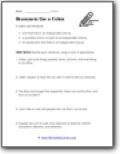###### Reasons to Use Them

Rewrite each sentence, using a colon if appropriate.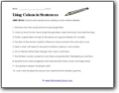###### Using Colons in Sentences

Correct each sentence by adding a colon where needed.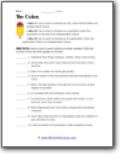###### The Rules

Add a colon to each sentence where needed. Write the number of the rule that applies on the line.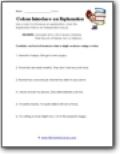###### Introduce an Explanation

Combine each set of sentences into a single sentence using a colon.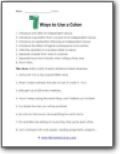###### 7 Ways to Use a Colon

1. Introduce a list after an independent clause; 2. Introduce a quotation that's not part of an independent clause;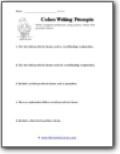###### Writing Prompts

Write 5 original sentences using colons. Follow the prompts below.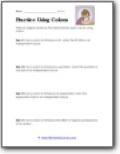###### Practice

Write an original sentence that demonstrates each rule.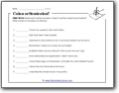###### Colon or Semicolon?

Read each sentence below. Does it use the correct punctuation? Write correct or incorrect on the line.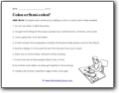###### Complete It

Complete each sentence by adding a colon or a semi-colon where needed.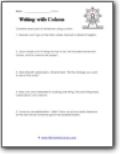###### Writing with Colons

Combine each pair of sentences using a colon.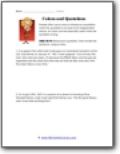###### Quotations

People often use a colon to introduce a quotation when the quotation is not part of an independent clause.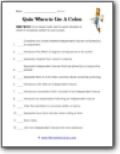###### Quiz: When to Use It

Put a check mark next to each situation in which it would be correct to use a colon.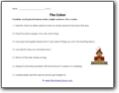###### Smash It

Combine each pair of sentences into a single sentence.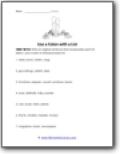###### Use a Colon with a List

Write an original sentence that incorporates each list below.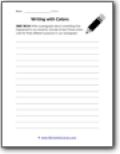###### Recently

Write a paragraph about something that happened to you recently.